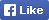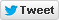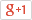Computer/Electronics Questions and Answers
C, C++, VC++, COM/DCOM, DLL and moreLoginSign upHome Electronics Computer Questions Member's Area News Site Map About

Write a program to find the sum of the even numbers upto the given range as well as sum of first 50 even numbers

Source Code

 1 #include 2 #include 3 int main (int argc, char *argv[]) 4 { 5 clrscr(); 6 int s=0,sr=0,n=0,i=0,c=0; 7 printf("\n Enter the range:"); 8 scanf("%d",&n); 9 while(c<=50) 10 { 11 if(i%2==0) 12 { 13 s+=i; 14 c++; 15 } 16 i++; 17 } 18 printf("\n The sum of 50 even numbers is: %d",s); 19 i=0; 20 c=0; 21 while(c<=n) 22 { 23 if(i%2==0) 24 { 25 sr+=i; 26 c++; 27 } 28 i++; 29 } 30 printf("\n The sum of %d even numbers is: %d",n,sr); 31 getch(); 32 }

Find More from our code collection
Armstrong number, binary number to a decimal number, bubble sort, decimal number to binary number, factorial of the given number factors, fibonacci numbers, HCF and LCM, matrix, mergesort, salary of the employee. palindrome, quadratic equation, star patterns, series etc. and much more...Return to Example Source Code